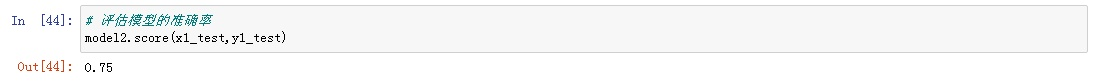### 如何使用python来进行回归分析

1.Linear Regression线性回归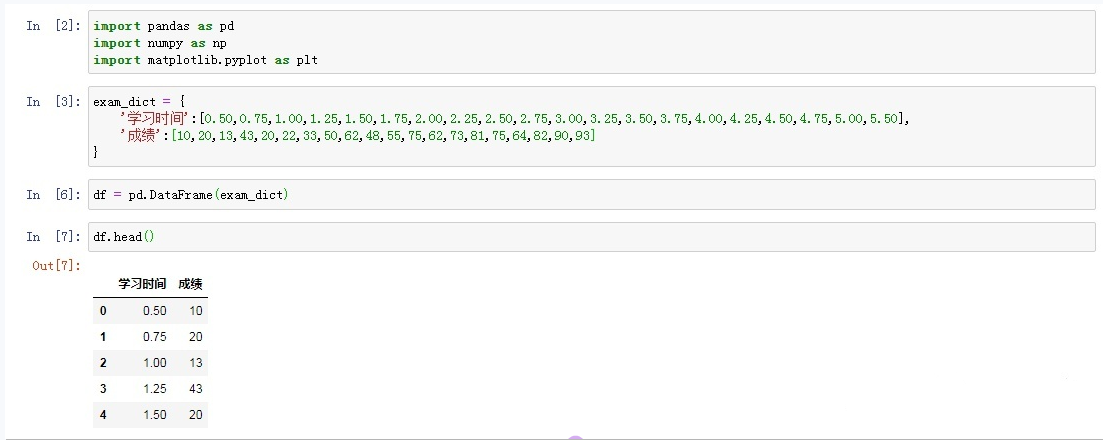先画一个散点图看下：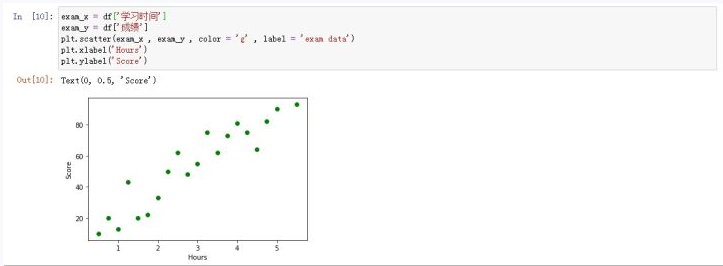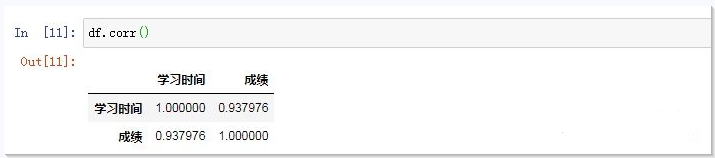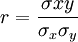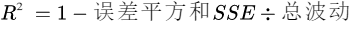2.Logistic Regression逻辑回归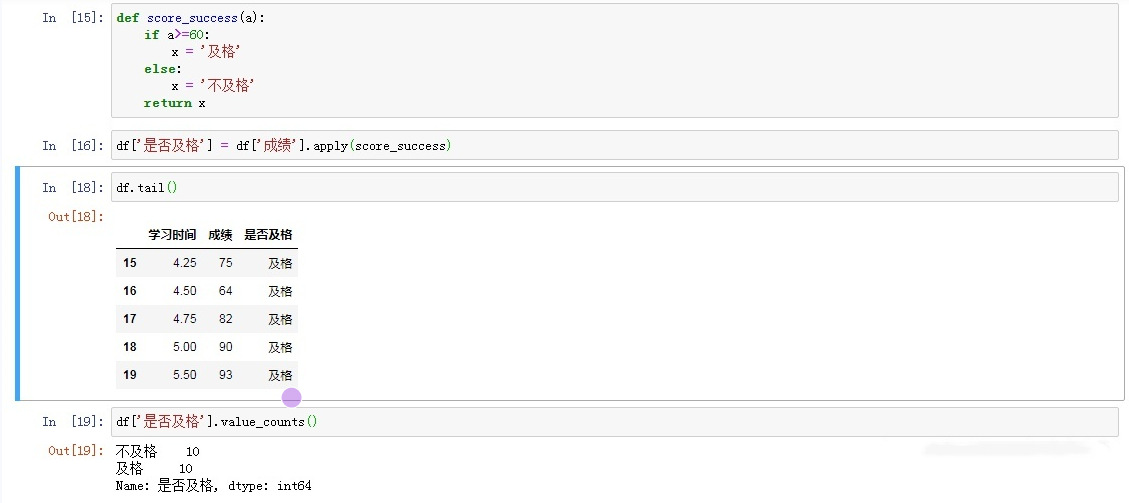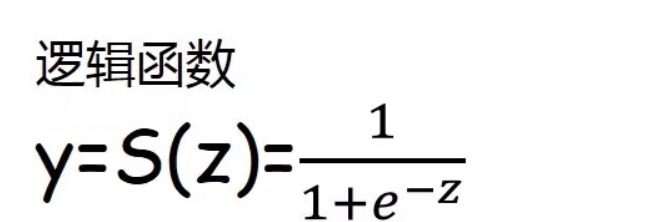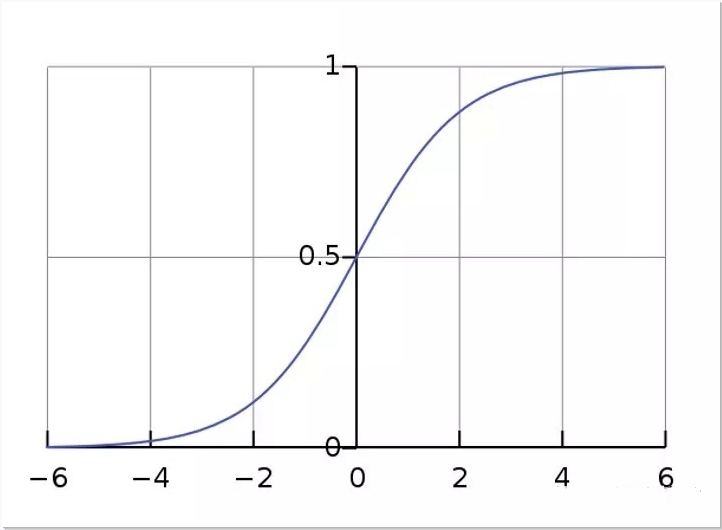1.线性回归的Python实现
① 提取特征x和标签y

``````# 特征features
exam_x = df.loc[:,'学习时间']
# 标签labels
exam_y = df.loc[:,'成绩']
``````
• 1
• 2
• 3
• 4

② 建立训练数据和测试数据

``````# 建立训练数据和测试数据
x_train , x_test , y_train , y_test = train_test_split(exam_x,exam_y,train_size = .8)
``````
• 1
• 2

train_size：训练数据占比，如果是整数的话就是样本的数量。
③ 输出数据大小

``````print('原始数据特征',exam_x.shape,
',训练数据特征',x_train.shape,
',测试数据特征',x_test.shape)
print('原始数据标签',exam_y.shape,
',训练数据标签',y_train.shape,
',测试数据标签',y_test.shape)
``````
• 1
• 2
• 3
• 4
• 5
• 6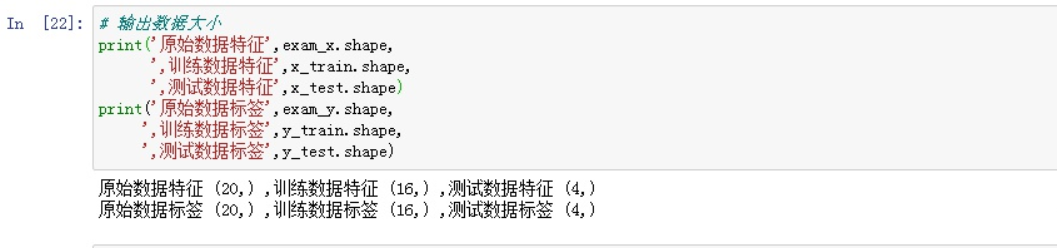④ 训练模型

``````# 1.导入线性回归
from sklearn.linear_model import LinearRegression
``````
• 1
• 2

``````# 2.创建模型：线性回归
model1 = LinearRegression()
``````
• 1
• 2

``````# 3.训练模型
x_train = x_train.values.reshape(-1,1)
x_test = x_test.values.reshape(-1,1)
model1.fit(x_train,y_train)
``````
• 1
• 2
• 3
• 4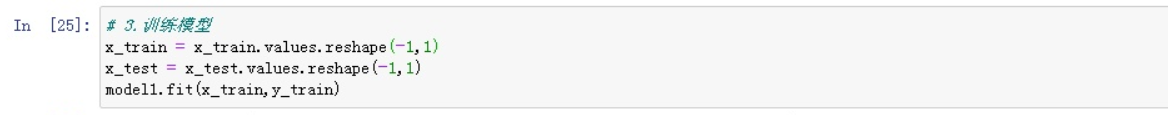``````# 截距
a = model1.intercept_
# 回归系数
b = model1.coef_
print('最佳拟合线：截距a = ',a,'回归系数b = ',b)
``````
• 1
• 2
• 3
• 4
• 5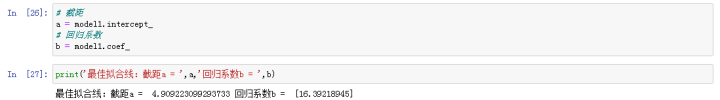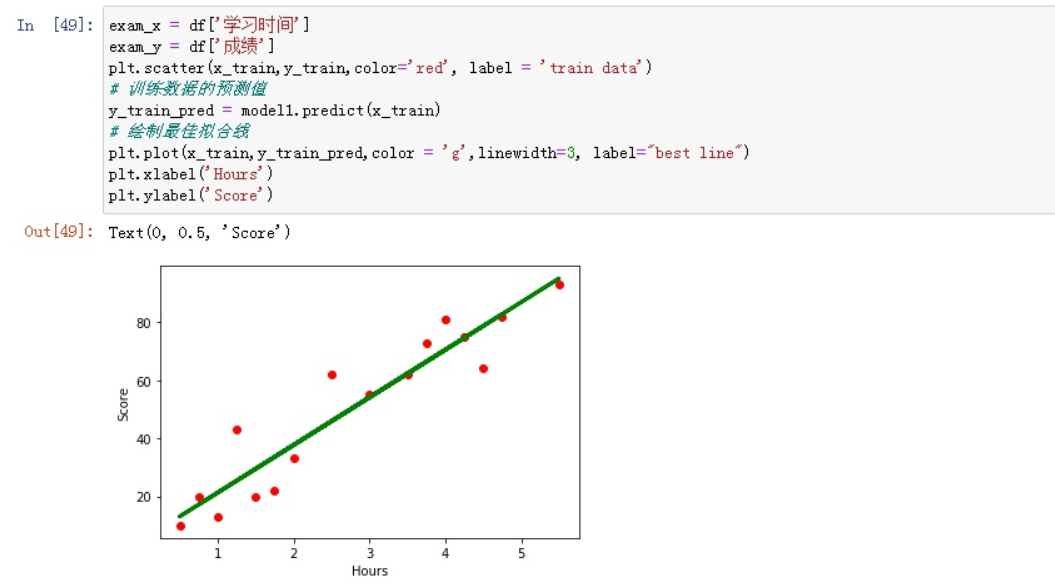⑤ 模型准确性评估

``````# 模型评估
model1.score(x_test , y_test)
``````
• 1
• 2

2.逻辑回归的Python实现

① 提取特征x和标签y

``````# 特征features
exam_x1 = df.loc[:,'学习时间']
# 标签labels
exam_y1 = df.loc[:,'是否及格']
``````
• 1
• 2
• 3
• 4

② 建立训练数据和测试数据

``````from sklearn.model_selection import train_test_split
# 建立训练数据和测试数据
x1_train , x1_test , y1_train , y1_test = train_test_split(exam_x1,exam_y1,train_size = .8)
``````
• 1
• 2
• 3

③ 输出数据大小：

``````# 输出数据大小
print('原始数据特征',exam_x1.shape,
',训练数据特征',x1_train.shape,
',测试数据特征',x1_test.shape)
print('原始数据标签',exam_y1.shape,
',训练数据标签',y1_train.shape,
',测试数据标签',y1_test.shape)
``````
• 1
• 2
• 3
• 4
• 5
• 6
• 7

④ 训练模型

``````# 训练模型
# 1.导入逻辑回归
from sklearn.linear_model import LogisticRegression
# 2.创建模型：逻辑回归
model2 = LogisticRegression()
# 3.模型训练
x1_train = x1_train.values.reshape(-1,1)
x1_test = x1_test.values.reshape(-1,1)
model2.fit(x1_train,y1_train)
``````
• 1
• 2
• 3
• 4
• 5
• 6
• 7
• 8
• 9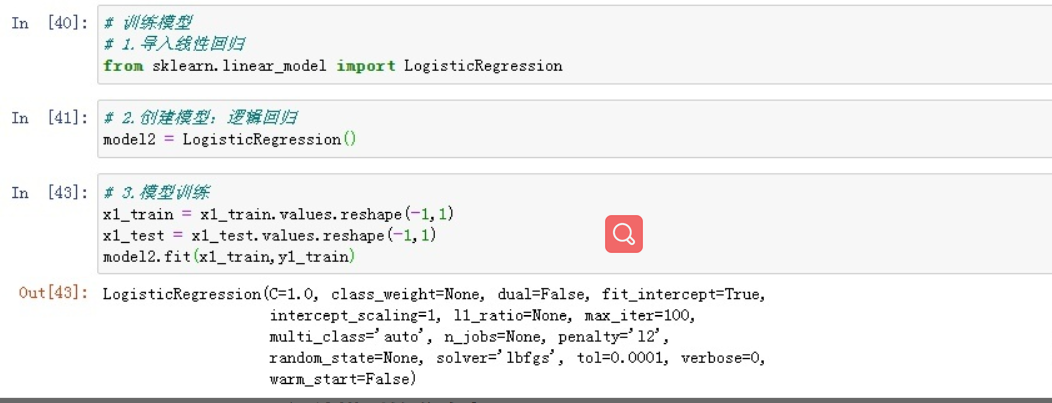⑤ 评估模型的准确率

``````# 评估模型的准确率
model2.score(x1_test,y1_test)
``````
• 1
• 2